## Mutation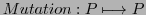In the first runs we do not use mutation. But, as one can easily see, in the absence of mutation will the development of the overall fitness be caught on a certain maximal level which can not be surpassed. Only the inclusion of mutation frees the algorithm from this 'trap' and allows a continuous approach to the theoretical maximum. It has to be discussed later how this phenomenon can be formally analyzed.

The result for the sum of all fitness values for 10 consecutive runs without mutation is shown below:

```run=10, [POP,FITNESS_ALLLOG]=gasimple(POP,l,p,n,run)

....

FITNESS_ALLLOG  =

2603.
2571.
2571.
2571.
2595.
2579.
2583.
2631.
2748.
2748.
```

The graphical representation of these numbers can be seen in figure 4.2.Here some more examples without mutation allowing longer periods with run = 50. The Y-axis in the following diagrams shows the gained fitness value as percentage of the theoretical possible maximum. In special cases where one simulates the fitness with the aid of a pre-defined fitness function like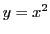this is possible. This - a bit artificial - situation allows direct analysis of the 'real' behavior of the GA.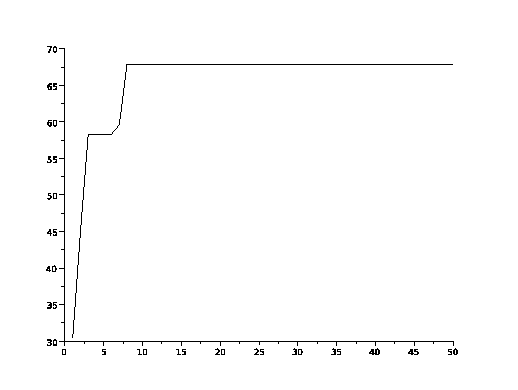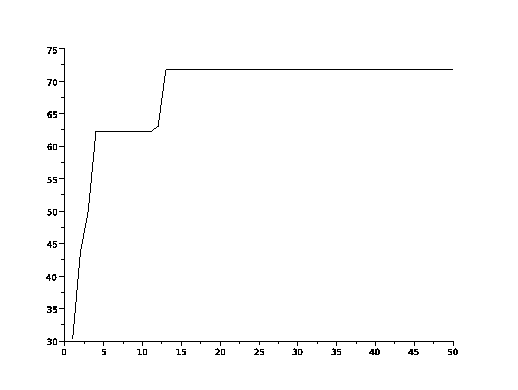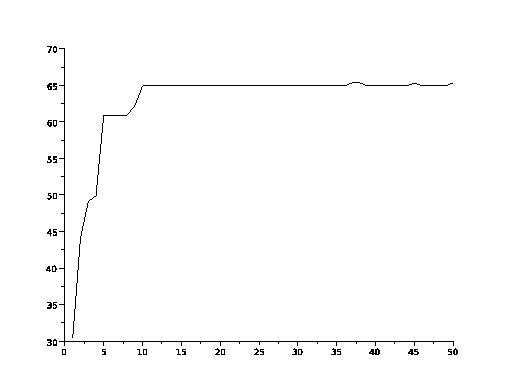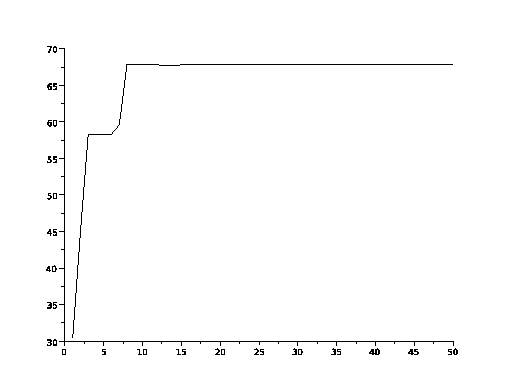One can easily observe the fact that the overall fitness value reaches quickly a certain upper level and then it is bound to this level. This can be understood as a hint that the available information in the gen pool can be limited with regard to the information needed to reach the theoretical maximum. If this hypothesis is true, then should the inclusion of a mutation operator change the gain in the overall fitness value significantly. This can be observed in the following diagrams.

These examples allow run periods with run = 80 with mutations every 10th cycle.

```MThreshold=10, [POP,FITNESS_ALLLOG]=gasimple(POP,l,p,n,run, MThreshold)
...
```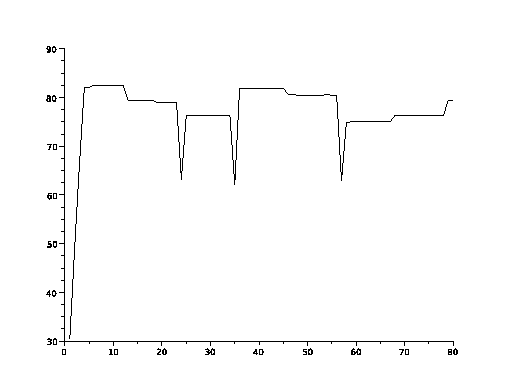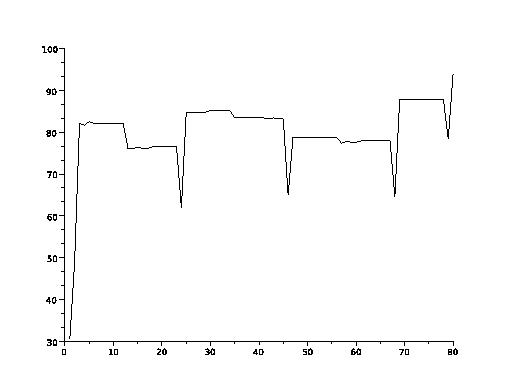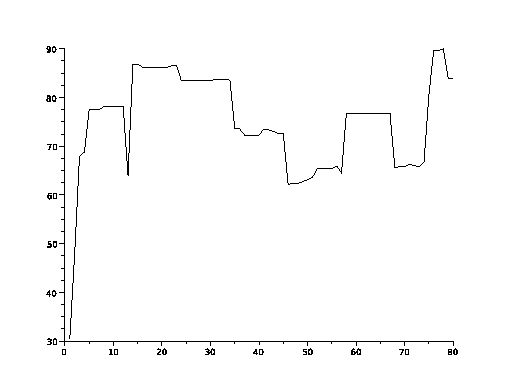If one compares the runs without mutation (cf. 4.3, 4.4, 4.5 and, 4.6, and 4.7) with those runs with mutations (cf. 4.8, 4.9,4.10,4.11,and 4.12), one can 'see' how the mutation-free runs reach some maximal level below the theoretical maximum and this level 'seems' to be a limit for the mutation-free runs. On the other side induce the diagrams with the mutation-active runs the impression that mutation can penetrate these mutation-free inherent levels.

This will be analyzed now.

Gerd Doeben-Henisch 2013-01-14